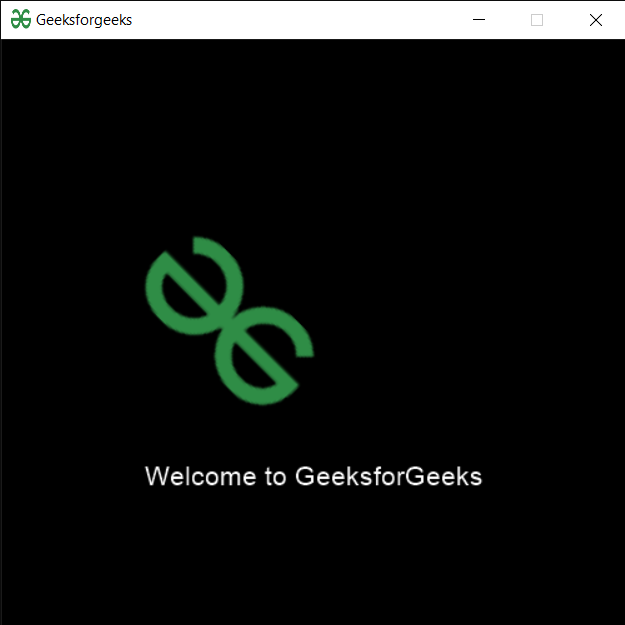# PYGLET – Updating Sprite

In this article we will see how we can update the sprite in PYGLET module in python. Pyglet is easy to use but powerful library for developing visually rich GUI applications like games, multimedia etc. A window is a “heavyweight” object occupying operating system resources. Windows may appear as floating regions or can be set to fill an entire screen (fullscreen). A sprite is an instance of an image displayed on-screen. Multiple sprites can display the same image at different positions on the screen. Sprites can also be scaled larger or smaller, rotated at any angle and drawn at a fractional opacity. Image is loaded with the help of image module of pyglet. Updating means to changing the properties of sprite, properties can be size, position etc.

We can create a window and sprite object with the help of commands given below

```# creating a window
window = pyglet.window.Window(width, height, title)

# creating a sprite object
sprite = pyglet.sprite.Sprite(img, x, y)
```

In order to create window we use `update` method with sprite object

Syntax : sprite.update(x=None, y=None, rotation=None, scale=None, scale_x=None, scale_y=None)

Argument : It takes optional argument i.e x, y co-ordinates (int), rotation (float), scale (float), horizontal scale (float), vertical scale (float)

Return : It returns None

Below is the implementation

 `# importing pyglet module  ` `import` `pyglet  ` `import` `pyglet.window.key as key ` `   `  `# width of window  ` `width ``=` `500` `   `  `# height of window  ` `height ``=` `500` `   `  `# caption i.e title of the window  ` `title ``=` `"Geeksforgeeks"` `   `  `# creating a window  ` `window ``=` `pyglet.window.Window(width, height, title)  ` `   `  `# text   ` `text ``=` `"Welcome to GeeksforGeeks"` `  `  `# creating label with following proeprties ` `# font = cooper ` `# position = 250, 150 ` `# anchor position = center ` `label ``=` `pyglet.text.Label(text,  ` `                          ``font_name ``=``'Cooper'``,  ` `                          ``font_size ``=` `16``,  ` `                          ``x ``=` `250``,   ` `                          ``y ``=` `150``,  ` `                          ``anchor_x ``=``'center'``,   ` `                          ``anchor_y ``=``'center'``) ` ` `  ` `  `# creating a batch  ` `batch ``=` `pyglet.graphics.Batch() ` ` `  `# loading geeksforgeeks image ` `image ``=` `pyglet.image.load(``'gfg.png'``) ` ` `  ` `  ` `  `# creating sprite object ` `# it is instance of an image displayed on-screen ` `sprite ``=` `pyglet.sprite.Sprite(image, x ``=` `200``, y ``=` `230``) ` `   `  `# on draw event  ` `@window``.event  ` `def` `on_draw():  ` `       `  `    ``# clear the window  ` `    ``window.clear()  ` `       `  `    ``# draw the label ` `    ``label.draw()  ` `     `  `    ``# draw the image on screen ` `    ``sprite.draw() ` `       `  `# key press event      ` `@window``.event  ` `def` `on_key_press(symbol, modifier):  ` `   `  `    ``# key "C" get press  ` `    ``if` `symbol ``=``=` `key.C:  ` `         `  `        ``# printng the message ` `        ``print``(``"Key : C is pressed"``) ` `         `  `# image for icon  ` `img ``=` `image ``=` `pyglet.resource.image(``"gfg.png"``)  ` ` `  `# setting image as icon  ` `window.set_icon(img)  ` ` `  `# updating the sprite ` `# x, y = 10, 300 ` `# rotation = 45 ` `# scale = 2 ` `sprite.update(``100``, ``300``, ``45``, ``2``) ` ` `  ` `  `    `  `# start running the application  ` `pyglet.app.run()  `

Output :Attention geek! Strengthen your foundations with the Python Programming Foundation Course and learn the basics.

To begin with, your interview preparations Enhance your Data Structures concepts with the Python DS Course.

My Personal Notes arrow_drop_upCheck out this Author's contributed articles.

If you like GeeksforGeeks and would like to contribute, you can also write an article using contribute.geeksforgeeks.org or mail your article to contribute@geeksforgeeks.org. See your article appearing on the GeeksforGeeks main page and help other Geeks.

Please Improve this article if you find anything incorrect by clicking on the "Improve Article" button below.

Article Tags :

Be the First to upvote.

Please write to us at contribute@geeksforgeeks.org to report any issue with the above content.